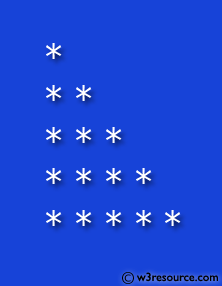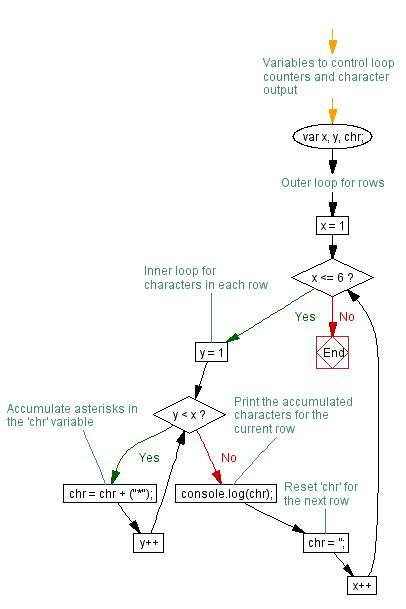# JavaScript: Construct a pattern, using a nested for loop

## JavaScript Conditional Statement and loops: Exercise-10 with Solution

Write a JavaScript program to construct the following pattern, using a nested for loop.

```*
* *
* * *
* * * *
* * * * *
```

Pictorial Presentation:Sample Solution:-

HTML Code:

``````
<!DOCTYPE html>
<html>
<head>
<meta charset=utf-8 />
<title>JS nested for loop example</title>
</head>
<body>

</body>
</html>
```
```

JavaScript Code:

``````
var x,y,chr;
for(x=1; x <=6; x++)
{
for (y=1; y < x; y++)
{
chr=chr+("*");
}
console.log(chr);
chr='';
}
```
```

Flowchart:Live Demo:

See the Pen javascript-conditional-statements-and-loops-exercise-10 by w3resource (@w3resource) on CodePen.

Improve this sample solution and post your code through Disqus

What is the difficulty level of this exercise?

﻿

## JavaScript: Tips of the Day

Convert tabs to spaces, where each tab corresponds to count spaces

Example:

```const tips_expandTabs = (str, count) => str.replace(/\t/g, ' '.repeat(count));
console.log(tips_expandTabs('\t\tw3resource', 4));
```

Output:

```"        w3resource"
```